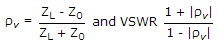Electronics and Communication Engineering - Microwave Communication - Discussion

48.

Assertion (A): Impedance measurement at microwave frequencies is done by finding SWR.

Reason (R): SWR and reflection coefficient depend on the characteristic impedance and load impedance.

 [A]. Both A and R are correct and R is correct explanation of A [B]. Both A and R are correct but R is not correct explanation of A [C]. A is correct but R is wrong [D]. A is wrong but R is correct

Explanation: